# 第三部分 数据结构

## 第十章 基本数据结构

### 栈

#### 基本操作（假设栈的容量为n$n$）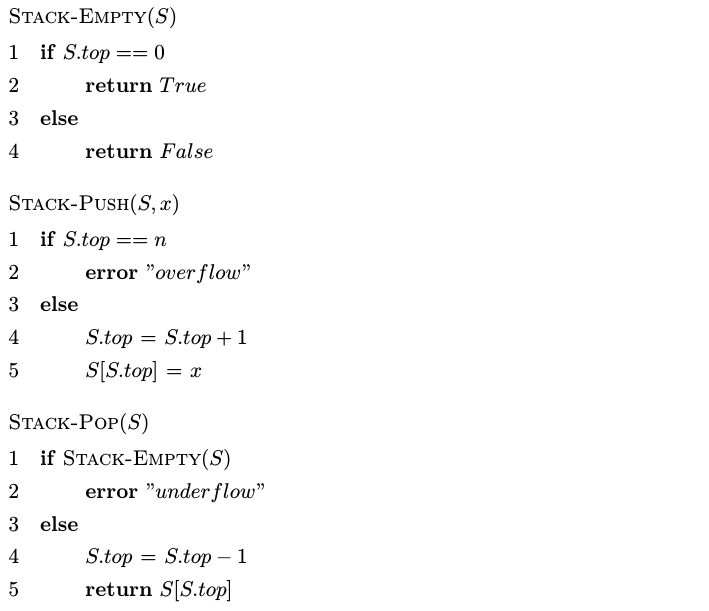### 队列

#### 基本操作（假设队列的容量为n$n$）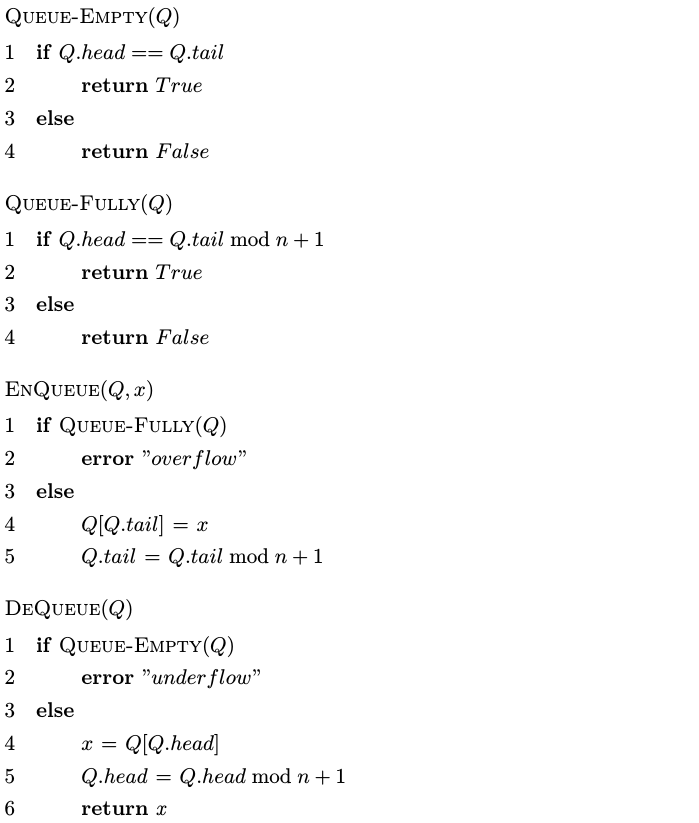### 链表

#### 基本操作（以双向链表为例）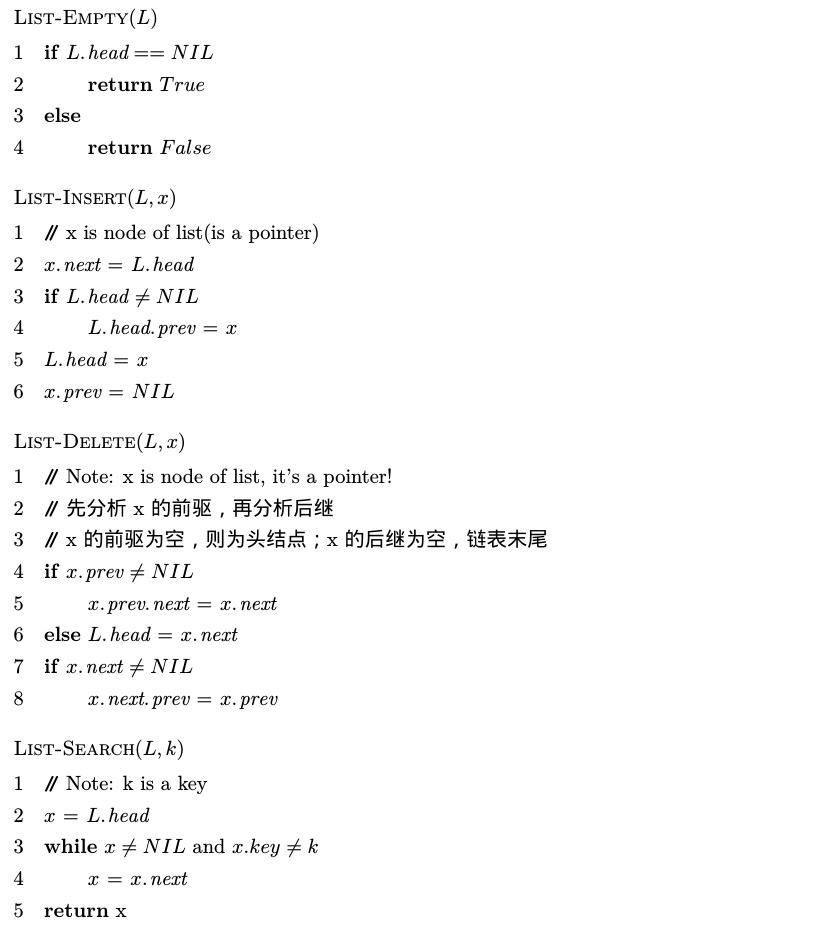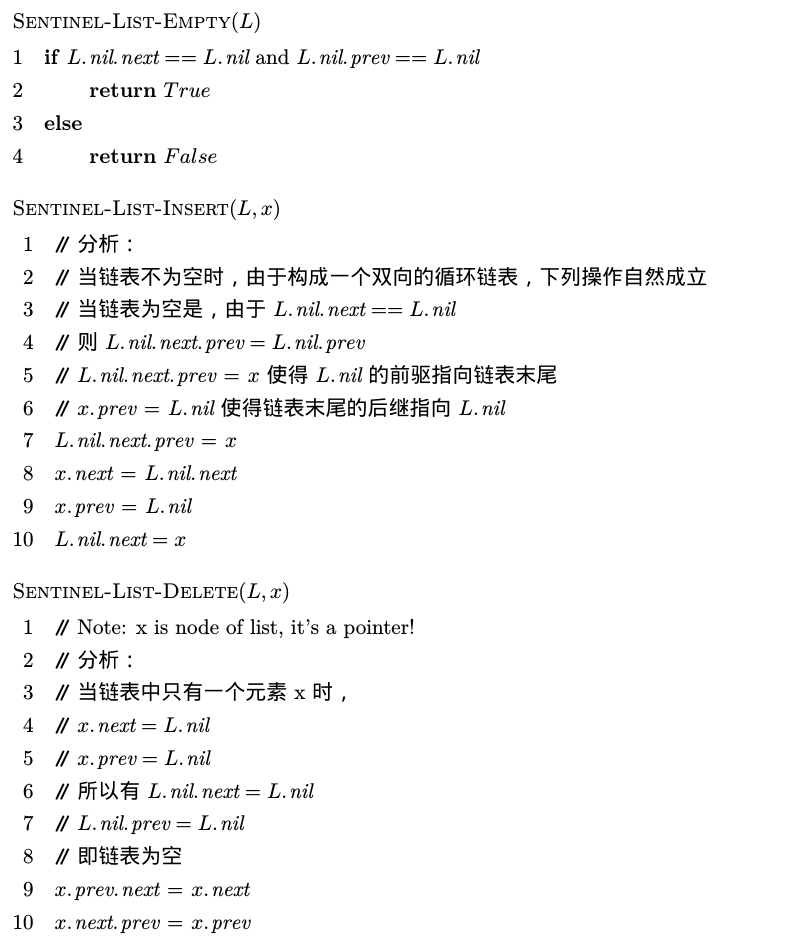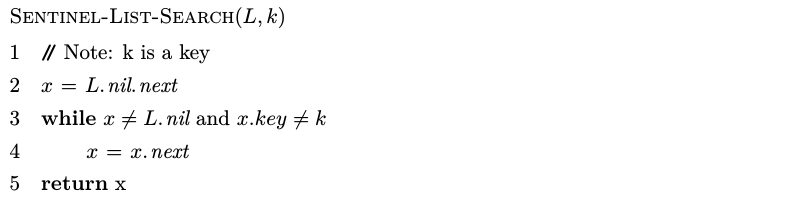## 第十一章 散列表

### 链接法散列的分析（散列函数满足简单均匀散列假设）

• 装载因子：给定一个能存放n$n$个元素的，具有m$m$个槽位的散列表T$T$T$T$的装载因子α$\alpha$即为其一个槽位中链表平均存储的元素个数。

• 链接法散列的最坏情况性能很差，如果所有关键字都散列到同一个槽中，则相当于一个长度为n$n$的链表，这时它的查找时间为Θ(n)$\Theta(n)$。当然，一般情况来说，散列表不会出现最坏情况，如果散列函数经过精心设计的话，其查找的期望时间一般都为常数。

• 查询操作 定理：在简单均匀散列的假设下，对于用链接法解决冲突的散列表，一次成功或不成功的查找平均时间均为Θ(1+α)$\Theta(1+\alpha)$

证明：

（查找不成功）

在简单均匀散列的假设下，任何尚未被存储在表中的关键字k$k$都等可能地被散列在m$m$个槽中的任何一个。当查找一个关键字k$k$不成功的情况下，查找的期望时间就是查找至链表T[h(k)]$T[h(k)]$末尾的期望时间，这个时间的期望长度为E[nh(k)]=α$E[n_{h(k)}] = \alpha$，所以，一次不成功的查找平均要检查α$\alpha$个元素，所需总时间（包含计算h(k)$h(k)$的时间）为Θ(1+α)$\Theta(1+\alpha)$

（查找成功）

怎么去分析这个问题？

由于我们查找成功，说明元素x$x$必定是这n$n$个元素中的一个且是等可能的。由散列表的插入性质（元素首先根据散列函数计算出关键字对应槽位，之后从对应槽位链表的表头插入），我们可知只有在元素x$x$之后的元素有可能插入在x$x$之前。而如果我们成功查找到x$x$，其实只需要查询x$x$所在链表，在x$x$之后插入到表中的期望元素数加1。我们假设元素xi$x_i$是第i$i$个元素，并且设ki=xi.key$k_i = x_i.key$，对关键字ki$k_i$kj$k_j$，定义指示器变量Xij=I{h(ki)=h(kj)}$X_{ij} = I\{h(k_i)=h(k_j)\}$。在简单均匀散列假设下，有Pr(h(ki)=h(kj))=1/m$Pr(h(k_i) = h(k_j)) = 1/m$，从而有E[Xij]=1/m$E[X_{ij}] = 1/m$。所以一次成功查找所检查的元素期望数目为

E[1ni=1n(1+j=i+1nXij)]=1ni=1n(1+j=i+1nE[Xij])=1ni=1n(1+j=i+1n1m)=1+1ni=1nj=i+1n1m=1+1nmi=1n(ni)=1+1nm[i=1nni=1ni]=1+1nm(n2n(n+1)2)=1+n12m=1+α2+α2n

所以，一次成功查找所需的全部时间（包括计算散列函数时间）为Θ(1+1+α2+α2n)=Θ(1+α)$\Theta(1+1+\frac{\alpha}{2}+\frac{\alpha}{2n}) = \Theta(1+\alpha)$

上述定理说明，查询操作平均需要常数时间。

• 插入操作：将散列到同一槽中的所有元素都放在同一链表中，且从表头插入元素，所以可知插入操作的最坏情况运行时间为O(1)$O(1)$

• 删除操作：删除操作需要根据使用的链表类型（单向链表和双向链表），以及元素输入类型（指针x$x$或关键字key$key$）不同分别考虑。

对于元素输入类型为关键字key$key$时，删除操作最坏情况运行时间为O(n)$O(n)$，平均运行时间与查找操作相同，为Θ(1+α)$\Theta(1+\alpha)$（假设满足简单均匀散列假设）；

对于元素输入类型为指针x$x$时，采用单向链表的散列表删除操作最坏情况运行时间为O(n)$O(n)$，平均运行时间与查找操作相同，为Θ(1+α)$\Theta(1+\alpha)$（假设满足简单均匀散列假设）；而双向链表最坏情况运行时间为O(1)$O(1)$

一般，将查询，插入，删除操作称为字典操作。散列表的字典操作平均情况都可以在O(1)$O(1)$时间内完成。

### 散列函数

#### 除法散列

h(k)=kmodm$h(k) = k \bmod m$

m$m$一般选择一个不太接近2的整数幂的素数。

#### 乘数散列

h(k)=m(kAmod1)$h(k) = \lfloor{m(kA\bmod 1)}\rfloor$

#### 全域散列

hab(k)=((ak+b)modp)modm$h_{ab}(k) = ((ak+b)\bmod p)\bmod m$

### 开放寻址法

h:U×{0,1,...,m1}{0,1,...,m1}

#### 线性探查

h(k,i)=(h(k)+i)modm;i=0,1,...,m1$h(k,i) = (h^\prime(k)+i)\bmod m; i=0,1,...,m-1$

h$h^\prime$为普通散列函数，在这里作为辅助散列函数。

#### 二次探查

h(k,i)=(h(k)+c1i+c2i2)modm;i=0,1,...,m1$h(k,i) = (h^\prime(k)+c_1i+c_2i^2)\bmod m; i=0,1,...,m-1$

h$h^\prime$为辅助散列函数，c1,c2$c_1,c_2$为正辅助常量。。

#### 双重探查

h(k,i)=(h1(k)+ih2(k))modm;i=0,1,...,m1$h(k,i) = (h_1(k)+ih_2(k))\bmod m; i=0,1,...,m-1$

h1,h2$h_1,h_2$均为辅助散列函数。为了能够查找整个散列表，值h2(k)$h_2(k)$必须要与m$m$互素。

#### 开放寻址散列的分析

• 给定一个装载因子为α=n/m<1$\alpha=n/m<1$的开放寻址散列表，并假设是均匀散列的，则对于一次不成功的查找，其期望探查次数至多为1/(1α)$1/(1-\alpha)$；由此可以推知插入一个元素至多需要做1/(1α)$1/(1-\alpha)$次探查，因为关键字被放入第一个遇到的空槽中。

• 定一个装载因子为α=n/m<1$\alpha=n/m<1$的开放寻址散列表，并假设是均匀散列的，则对于一次不成功的查找，其期望探查次数至多为1αln11α$\frac{1}{\alpha}ln\frac{1}{1-\alpha}$；由此可知，删除一个元素至多需要1αln11α$\frac{1}{\alpha}ln\frac{1}{1-\alpha}$次探查。

假设散列表是半满α=n/m=50%$\alpha=n/m=50\%$的，一次不成功查找的平均探查次数至多为1/(10.5)=2$1/(1-0.5) = 2$，一次成功查找的平均探查次数至多为1/0.5ln(1/(10.5))<1.387$1/0.5ln(1/(1-0.5))<1.387$

假设散列表是α=n/m=90%$\alpha=n/m=90\%$满的，一次不成功查找的平均探查次数至多为1/(10.9)=10$1/(1-0.9) = 10$，一次成功查找的平均探查次数至多为1/0.9ln(1/(10.9))<2.559$1/0.9ln(1/(1-0.9))<2.559$

### 完全散列（perfect hashing）

#### 完全散列的几条定理

• 定理1：如果从一个全域散列函数类中随机选出散列函数h$h$，将n$n$个关键字存储在一个大小为m=n2$m=n^2$的散列表中，那么表中出现冲突的概率小于1/2$1/2$

证明：n$n$个关键字，则有(n2)$\left(\begin{array}{} n\\2 \end{array}\right)$对关键字可能发生冲突，如果散列函数从全域散列函数类中选出，则每对关键字发生冲突的概率为1/m$1/m$，设X$X$是一个统计冲突次数的随机变量，当m=n2$m=n^2$时，期望的冲突次数为

E[X]=(n2)1m=n(n1)21n2<12

• 定理2：如果从某一个全域散列函数类中随机选出散列函数h$h$，用它将n$n$个关键字存储在一个大小为m=n$m=n$的散列表中，则有E[m1j=0n2j]<2n$E[\sum_{j=0}^{m-1}n_j^2]<2n$，这里nj$n_j$为散列到槽j$j$中的关键字数。

证明：我们有数学恒等式：a2=a+2(a2)$a^2 = a+2\left(\begin{array}{}a\\2\end{array}\right)$，于是有

E[j=0m1n2j]=E[j=0m1(nj+2(nj2))]=E[j=0m1nj]+2E[j=0m1(nj2))]=n+2E[j=0m1(nj2)]

其中m1j=0(nj2)$\sum_{j=0}^{m-1}\left(\begin{array}{} n_j\\2\end{array}\right)$是散列表中发生冲突的关键字的总对数，根据全域散列性质，这一和式期望至多为
(n2)1m=n(n1)2m=n12

所以，有
E[j=0m1n2j]n+2n12=2n1<2n

• 推论1：如果从某一全域散列函数类中随机选出散列函数h$h$，用它将n$n$个关键字存储在一个大小m=n$m=n$的散列表中，并将每一个二级散列表的大小设置为mj=n2j(j=0,1,...,m1)$m_j=n_j^2(j=0,1,...,m-1)$，则在一个完全散列方案中，存储在所有二次散列表中所需的存储总量的期望值小于2n$2n$

证明：

mj=n2jE[j=0m1mj]=E[j=0m1n2j]<2n

• 推论2：如果从某一个全域散列函数类中随机选出散列函数h$h$，用它将n$n$个关键字存储到一个大小为m=n$m=n$的散列表中，并将每个二级散列表的大小置为mj=n2j(j=0,1,...,m1)$m_j=n_j^2(j=0,1,...,m-1)$，则用于存储所有二级散列表的存储总量等于或大于4n$4n$的概率小于1/2$1/2$

证明：由马尔科夫不等式Pr{Xt}E[X]/t$Pr\{X\geq t\}\leq E[X]/t$可推知

Pr{j=0m14n}E[m1j=0mj]4n<2n4n=1/2

推论2中可以看出，只需从全域散列函数类中随机选出几个散列函数，尝试几次就可以快速地找到一个所需存储量较为合理的函数。